# Rampage

In this episode, a shooter attacks the FBI office and Charlie and the FBI team try to find out why.

## Inequality Bounding

At the beginning of the episode, Charlie explains how to understand the connecting between a child molestor and his victim by looking at the intersection of the areas that each person uses. A very simple example is assuming that both the victim and perpetrator move in circles of radii r1 and r2 centered at (x1,y1) and (x2,y2).

Activity 1: Finding the area of the intersection of two circles.

The following steps would allow you to find a formula to compute the area intersection of  the intersection of two circles, say  with radii r1,r2 and centered at (x1,y1) and (x2,y2).

1. Draw a picture of two circles with radii r1 and r2, and centerd at C1=(x1,y1) and C2=(x2,y2). Draw the circles so the area of intersection is not zero. Also, name the intersection points I1 and I2.
2. What is the distance between P1 and P2? Call this distance D.
3. Find the angles I1C1I2 and I1C2I2. [Hint: Use the law of cosines to get I1C1C2 and notice that
I1C1I2=2I1C1C2. Do likewise for I1C2I2]
4. Make sure the angles are expressed in radians.
5. Find the area of the circular sectors I1C1I2 and I1C2I2 [Hint: Recall that the area of a circular sector is given by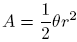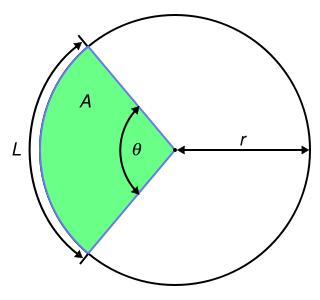6. Find the area of the triangles I1C1I2 and I2C2I2.
7. Deduce a formula for the area of the intersection of the two circles.
8. Agoat is tied to a point on the perimeter of a circular field of radius 8 ft. The length of the rope is 2 ft. What is the area of the field accessible to the goat?

## Brownian Motion

Analizing the path taken by the shooter in the FBI building, Charlie noticed that the path describes was a Brownian path. Basically, Brownian motion refers to the random movement of a particle in a fluid. A nice computer simulation can be found in this link. This concept was introduced by the Scottish botanist Robert Brown (1773 - 1858)  who used his microscope to look at pollen floating in water. He noticed that the pollen grains moved in a very unpredictable way, and the same occurred with other very small particles. He assumed that the movement was due to physical causes (and in fact it is now know that this unpredictable movement is due to the bombardment of the water molecules). Albert Einstein used Brownian motion to (indirectly) confirm the existance of molecules and atoms, while the mathematician Louis Bachelier used Brownian motion to analyze the stock market (stock options) in the first paper to used advanced mathematics to study finance.

## Loop-erased Random Walk

Trying to understand the perpetrator's path in the FBI rampage, Charlie used the concept of loop-erased random walk. To describe the concept, suppose one has a graph G and selects a path in G; that is, a collection of  vertices v1, v2, . . ., vn such that two consecutive vertices form an edge in G. This path may contain  loops (a path where the initial and end point are the same). A loop-erased random walk in G is the a path where the loops have been removed chronologically.

Activity 2
1. Consider the picture below the picture above, suppose a path is given by 1,3,4,5,5.  What would be the loop-erased walk corresponding to that path?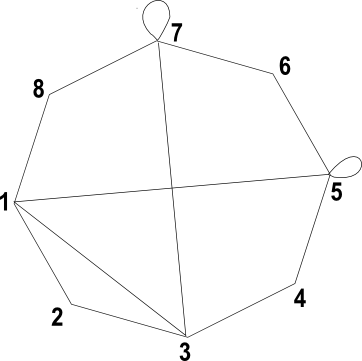2. What if the path is 1,2,3,4,5,6,7,7,3,7?

3. A spanning tree of a graph G is a tree (a graph with no cycles) whose vertex set is the same as G’s. How many spanning trees does the graph above have?

## Tesseract and Schlegel Diagrams

As pointed out in the episode, a tesseract is a 3-dimensional representation of the 4 dimensional cube. A tesseract is an example of a Schlegel diagram, invented by the German mathematician Victor Schlegel (1843-1905). A Schlegel diagram encodes a d-dimensional polytope (a polyhedron in more than 3 dimensions) into a an object of dimension d - 1. To draw a Schlegel diagram, follow the following steps:

1. Pick a face F and a point P "outside" the center of F (see picture below).
2. Draw lines between the point P and all the vertices of the figure.
3. Mark the points where these lines intersect F.
4. Imagine F is made out of rubber bands, and move the vertices to their intersection in F. The edges outside F will correspond to lines connecting the intersections in F.

The resulting picture is the Schlegel diagram. The example below describes the construction of the Schlegel diagram of the tetrahedron. Here the edges outside F are A, B, and C, and they correspond to A, B, and C, respectively, in the red figure. Notice that the actual Schlegel diagram depends on our choice of F. In the case of the tethrahedron, since all the faces have the same shape, the choice of F is irrelevant.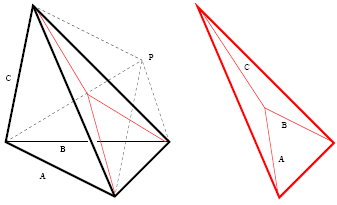These are the Schlegel diagrams for the platonic solids, appearing in Schlegel's original paper.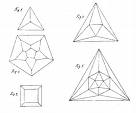Activity 3
• Draw the Schlegel diagram of a 3-dimensional cube.

• Draw the Schlegel diagram of the triangular prism depicted below. First use one of the triangular faces, and then use one of the rectangular ones. You should get different diagrams.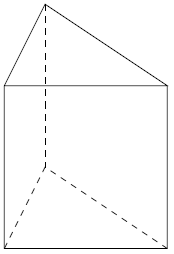• Convince yourself that a tessaract is in fact the Schlegel diagram of the 4-dimensional cube. Looking back at 1. above may help.